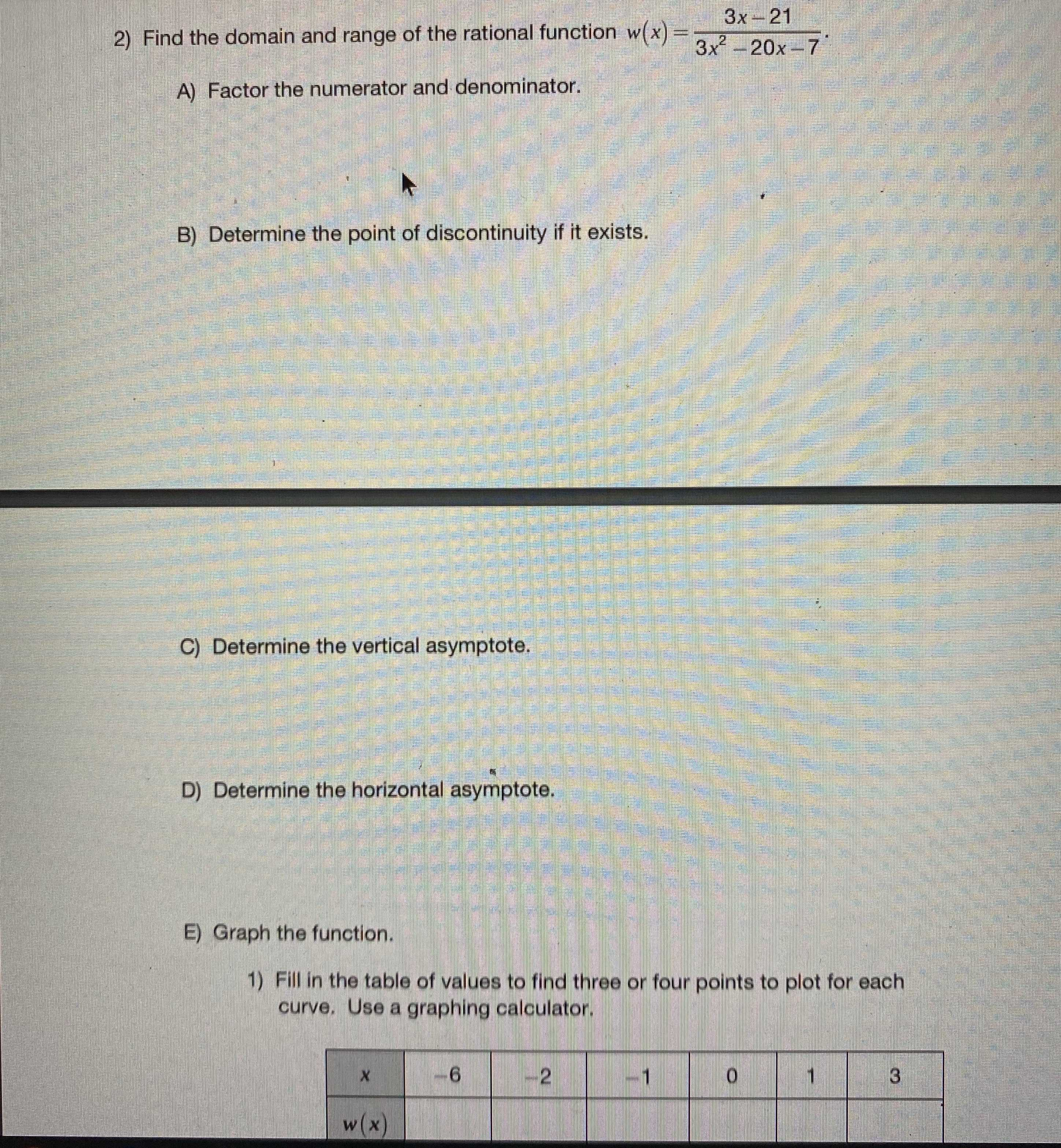### ¿Todavía tienes preguntas de matemáticas?

Pregunte a nuestros tutores expertos
Algebra
Pregunta2) Find the domain and range of the rational function $$w ( x ) = \frac { 3 x - 21 } { 3 x ^ { 2 } - 20 x - 7 }$$

A) Factor the numerator and denominator. B) Determine the point of discontinuity if it exists. C) Determine the vertical asymptote. D) Determine the horizontal asymptote. E) Graph the function. 1) Fill in the table of values to find three or four points to plot for each curve. Use a graphing calculator.

Domain $$\{ x \in R : x \neq - \frac { 1 } { 3 }$$and $$x \neq 7 \}$$

Range $$\{ w \in R : w \neq 0$$ and $$w \neq \frac { 3 } { 22 } \}$$

C) $$x= -\frac{1}{3}$$

D) y=0

E)

Solución
View full explanation on CameraMath App.